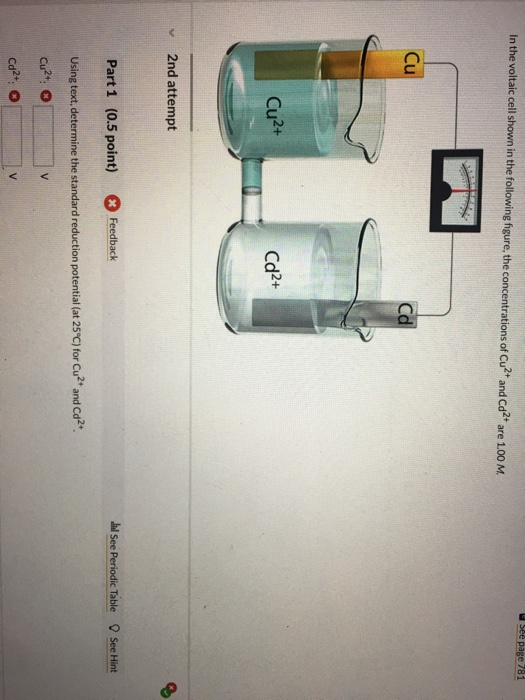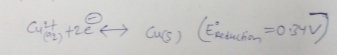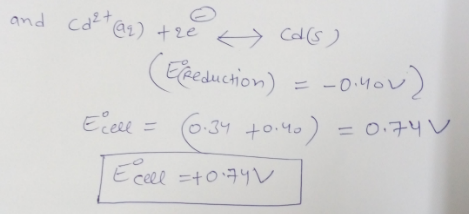# Question & Answer: In the voltaic cell shown in the following figure, the concentrations of Cu^2+ and Cd^2+ are…..In the voltaic cell shown in the following figure, the concentrations of Cu^2+ and Cd^2+ are 1.00 M. Using text, determine the standard reduction potential (at 25 degree C) for Cu^2+ and Cd^2+. Cu^2+: V Cd^2+: V